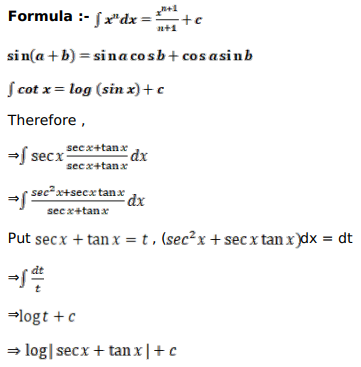# Mark against the correct answer in each of the following:

Question:

Mark $(\sqrt{ })$ against the correct answer in each of the following:

$\int \sec x d x=?$

A. $\log |\sec x-\tan x|+C$

B. $-\log |\sec x+\tan x|+C$

C. $\log |\sec x+\tan x|+C$

D. none of these

Solution: